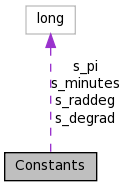# Constants Class Reference

`#include <Constants.h>`

Collaboration diagram for Constants:[legend]

List of all members.

## Static Public Member Functions

static double sinDeg (double x)
static double cosDeg (double x)
static double asinDeg (double x)
static double acosDeg (double x)
static double arcminToDeg (double aArcmin)

## Static Public Attributes

static const long double s_pi = 3.141592653589793238462643
static const long double s_raddeg = Constants::s_pi / 180.0
static const long double s_degrad = 180.0 / Constants::s_pi
static const long double s_minutes = 60.0

## Private Member Functions

Constants ()
~Constants ()
Constants (Constants const &)
Constantsoperator= (Constants const &)

## Detailed Description

Definition at line 12 of file Constants.h.

## Constructor & Destructor Documentation

 Constants::Constants ( ) ` [inline, private]`

Definition at line 38 of file Constants.h.

 Constants::~Constants ( ) ` [inline, private]`

Definition at line 39 of file Constants.h.

 Constants::Constants ( Constants const & ) ` [private]`

## Member Function Documentation

 static double Constants::acosDeg ( double x ) ` [inline, static]`

Definition at line 25 of file Constants.h.

 static double Constants::arcminToDeg ( double aArcmin ) ` [inline, static]`

Definition at line 32 of file Constants.h.

References s_minutes.

 static double Constants::asinDeg ( double x ) ` [inline, static]`

Definition at line 24 of file Constants.h.

Referenced by Object::computeRACorrection(), and Object::haversineDistance().

 static double Constants::cosDeg ( double x ) ` [inline, static]`

Definition at line 23 of file Constants.h.

Referenced by Object::computeRACorrection(), and Object::haversineDistance().

 static double Constants::degToArcmin ( double aDeg ) ` [inline, static]`

Definition at line 28 of file Constants.h.

References s_minutes.

 Constants& Constants::operator= ( Constants const & ) ` [private]`
 static double Constants::sinDeg ( double x ) ` [inline, static]`

Definition at line 22 of file Constants.h.

Referenced by Object::computeRACorrection(), and Object::haversineDistance().

## Member Data Documentation

 const long double Constants::s_degrad = 180.0 / Constants::s_pi` [static]`

Definition at line 17 of file Constants.h.

Referenced by acosDeg(), and asinDeg().

 const long double Constants::s_minutes = 60.0` [static]`

Definition at line 18 of file Constants.h.

Referenced by arcminToDeg(), degToArcmin(), and Object::gcDistanceMins().

 const long double Constants::s_pi = 3.141592653589793238462643` [static]`

Definition at line 15 of file Constants.h.

 const long double Constants::s_raddeg = Constants::s_pi / 180.0` [static]`

Definition at line 16 of file Constants.h.

Referenced by cosDeg(), and sinDeg().

The documentation for this class was generated from the following files:
Generated on Mon Oct 4 10:40:29 2010 for Matching.kdevelop by1.6.3